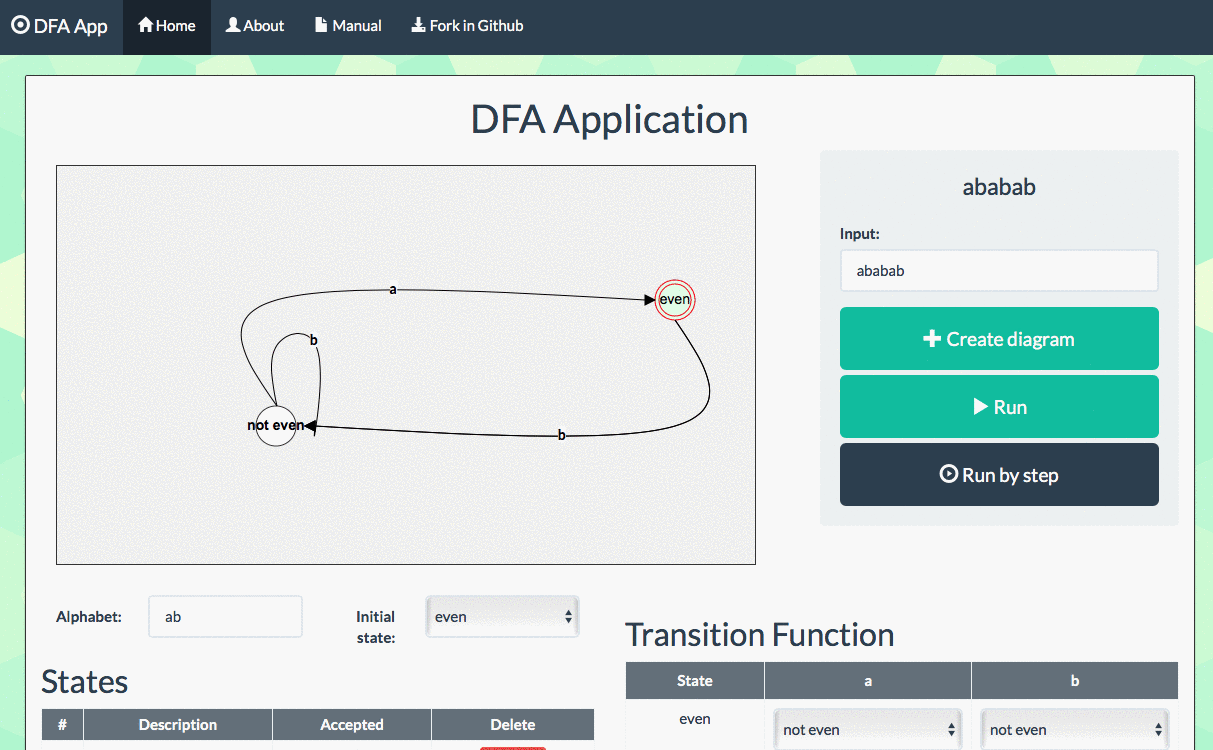Back

# DFADeterministic Finite Automata (DFA) application to test validity of inputs against an alphabet and transition function.

A Deterministic Finite Automata (DFA) is defined as a 5-tuple $M = (Q, \Sigma, q_0, F, \delta)$, where:

• $Q$: is a finite set of states
• $\Sigma$: is an alphabet
• $q_0$: is an initial state
• $F$: is a set of accepted states
• $\delta$: is a transition function which determines how to jump from one state to another.